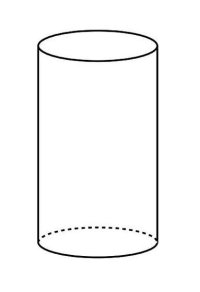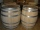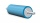# Cylinder - A&V

The cylinder has a volume 1287. The base has a radius 10. What is the area of surface of the cylinder?

Result

A =  885.7

#### Solution:

$r = 10 \ \\ V = \pi r^2 v \ \\ v = \dfrac{ 1287}{\pi r^2} = 4.097 \ \\ A = 2S_1 + S_2 = 2\pi r^2 + 2\pi r \cdot v = 2\pi \cdot 10 \cdot (10+4.097) = 885.7$Our examples were largely sent or created by pupils and students themselves. Therefore, we would be pleased if you could send us any errors you found, spelling mistakes, or rephasing the example. Thank you!

Leave us a comment of this math problem and its solution (i.e. if it is still somewhat unclear...):Be the first to comment!## Next similar math problems:

1. The cylinder 2Find the volume and the lateral area of a cylinder of height 12 inches and a base radius of 4 inches.
2. Secret treasureScouts have a tent in the shape of a regular quadrilateral pyramid with a side of the base 4 m and a height of 3 m. Determine the radius r (and height h) of the container so that they can hide the largest possible treasure.
3. Ratio of volumesIf the heights of two cylindrical drums are in the ratio 7:8 and their base radii are in the ratio 4:3. What is the ratio of their volumes?
4. Height as diameter of baseThe rotary cylinder has a height equal to the base diameter and the surface of 471 cm2. Calculate the volume of a cylinder.
5. Cube into cylinderIf we dip a wooden cube into a barrel with a 40cm radius, the water will rise 10 cm. What is the size of the cube edge?
6. Two cylindersTwo cylinders are there one with oil and one with an empty oil cylinder has no fixed value assume infinitely. We are pumping out the oil into an empty cylinder having radius =1 cm height=3 cm rate of pumping oil is 9 cubic centimeters per sec and we are p
7. Metal tubeCalculate the metal tube mass 8dm long with the outer radius 5cm and the inner radius 4.5cm and 1cm3 of this metal is 9.5g.
8. BottleA company wants to produce a bottle whose capacity is 1.25 liters. Find the dimensions of a cylinder that will be required to produce this 1.25litres if the hight of the cylinder must be 5 times the radius.
9. A cylindrical tankA cylindrical tank can hold 44 cubic meters of water. If the radius of the tank is 3.5 meters, how high is the tank?
10. CylinderThe 1.8m cylinder contains 2000 liters of water. What area (in dm2) of this container is the water?
11. Cylinder and its circumferenceIf the height of a cylinder is 4 times its circumference c, what is the volume of the cylinder in terms of its circumference, c?
12. WinchThe steel rope has a diameter of 6mm and a length of 20m. We are winding on drum width 60mm, starting diameter 50mm. What is the final diameter after winding?
13. Axial cut of a rectangleCalculate the volume and surface of the cylinder whose axial cut is a rectangle 15 cm wide with a diagonal of 25 cm long.
14. Iron densityCalculate the weight of a 2 m long rail pipe with an internal diameter of 10 cm and a wall thickness of 3 mm. The iron density is p = 7.8 g/cm3.
15. Steel tubeThe steel tube has an inner diameter of 4 cm and an outer diameter of 4.8 cm. The density of the steel is 7800 kg/m3. Calculate its length if it weighs 15 kg.
16. Diameter of a cylinderI need to calculate the cylinder volume with a height of 50 cm and a diameter of 30 cm.
17. The potThe pot is a cylinder with a volume of V = 7l and an inner diameter of d = 20cm. Find its depth.